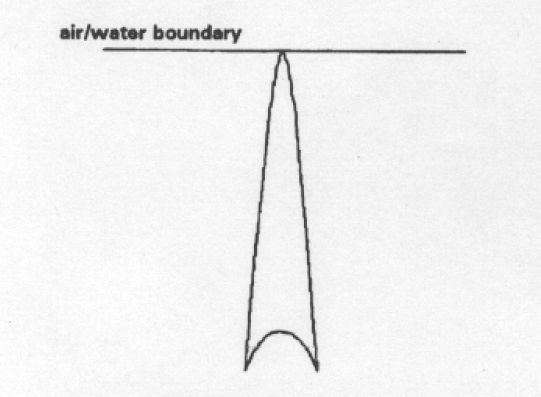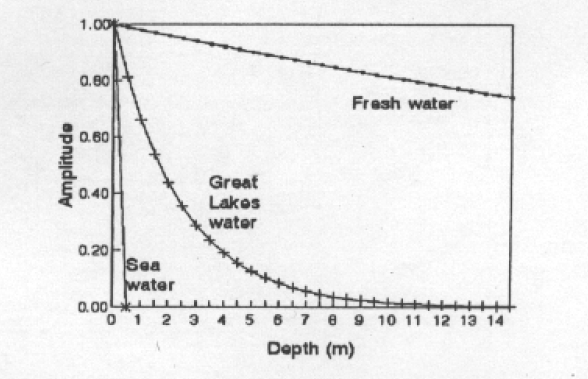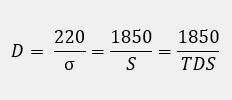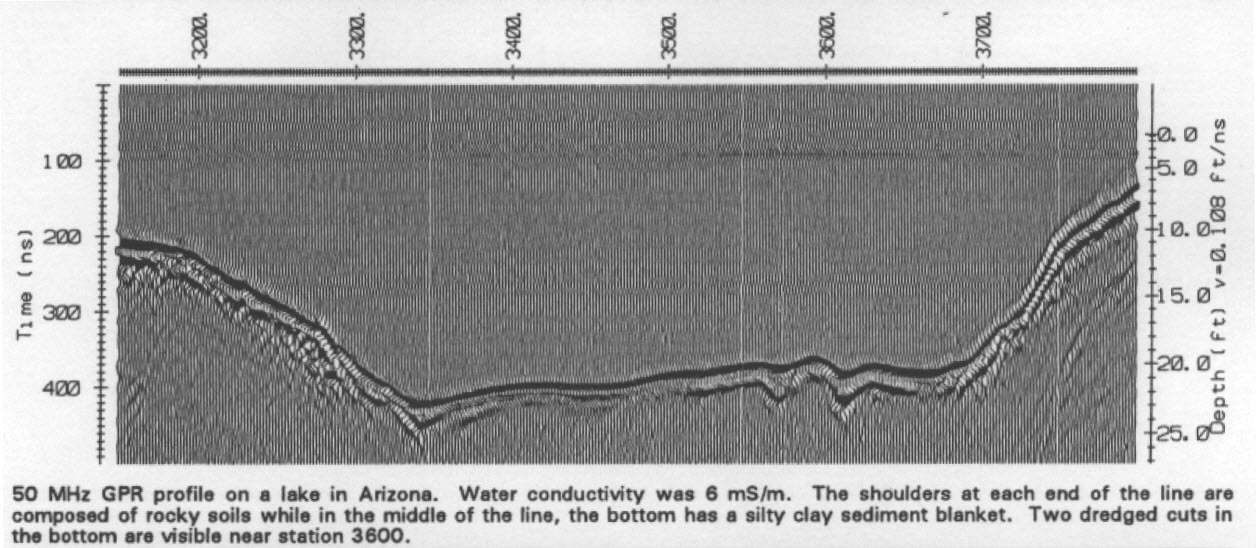GPR Sounding in Water – EKKO UpdateX

# GPR Sounding in Water – EKKO Update

G
round penetrating radar is often used to map water depth and sub-bottom stratigraphy. Such applications defy the myth that GPR does not work in water.

Fresh water is an ideal environment for ground penetrating radar. The propagation velocity is very slow so that high resolutions can be achieved. Typically, the propagation velocity is about 1/9 the speed of light. For example, the wavelength in air at 100 MHz is 3m whereas in water it is about 33 cm.

Refractive focusing at the air-water interface generates a very narrow beam of energy into the subsurface. Figure 1 illustrates the ± 7° beam width in water.Figure 1
GPR beam pattern in water

Sounding in water is limited by the electrical conductivity of the water. Water conductivity is controlled by the salinity or totally dissolved solids in the water.

A rough guide to GPR penetration depth in water is:

D = 40/α meters

Where the attenuation coefficient, α, is related to electrical conductivity, σ (in mS/m), by:

α = 0.18 σ dB/m

The drop off in signal amplitude versus depth associated with water conductivity is illustrated in Figure 2. Fresh water shows low attenuation of signal whereas sea water eliminates the signal in a very short distance.Figure 2
GPR signal attenuation vs depth water

Quite often one does not have water conductivity but other measures such as salinity or total dissolved solids. A rough guide to estimating conductivity of the water is to use the following relationships:

σ = 0.12 x S mS/m

σ = 0.12 x TDS mS/m

Where S is a salinity in parts per million and TDS is the total dissolved solids in the milligrams per liter. Combining the results together one gets that:An example of GPR profiling on a lake in Arizona is shown in Figure 3. The water conductivity was 6mS/m.

The survey was carried out by simply hanging the transmitting and receiving antennas over either side of a boat. This quick survey illustrates the utility of GPR in surveying water bottom.Figure 3:50 MHz GPR profile on lake in Arizona
Water conductivity was 6mS/m. The shoulders at each end of the line are composed of rocky soils while in the middle of the line, the bottom has a clay sediment blanket. Two dredged cuts in the bottom are visible near station 3600.
﻿

﻿